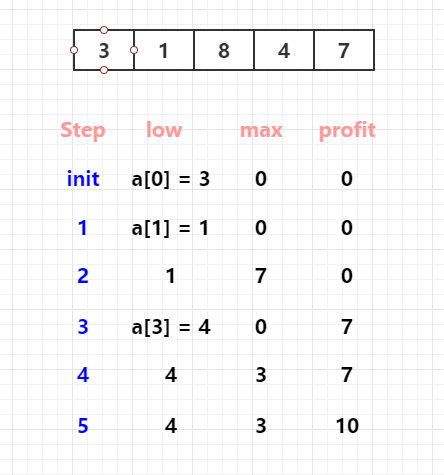# leetcode -  Best Time to Buy and Sell Stock II

2020/02/16

## 题目描述

Say you have an array for which the ith element is the price of a given stock on day i.
Design an algorithm to find the maximum profit.
You may complete as many transactions as you like (i.e., buy one and sell one share of the stock multiple times).

``````Example 1:

Input: [7,1,5,3,6,4]
Output: 7
Explanation: Buy on day 2 (price = 1) and sell on day 3 (price = 5), profit = 5-1 = 4.
Then buy on day 4 (price = 3) and sell on day 5 (price = 6), profit = 6-3 = 3.

Example 2:

Input: [1,2,3,4,5]
Output: 4
Explanation: Buy on day 1 (price = 1) and sell on day 5 (price = 5), profit = 5-1 = 4.
Note that you cannot buy on day 1, buy on day 2 and sell them later, as you are
engaging multiple transactions at the same time. You must sell before buying again.
``````

## `解法1`

``````@Test
public void question122(){
int[] prices = {7, 1, 5, 3, 6, 4};
int ret = ArrayProblems.p122(prices);
Assert.assertEquals(ret, 7);
}

public class ArrayProblems {
public static int p122(int[] prices) {
int amount = 0;
for (int i = 1; i < prices.length; i++){
if (prices[i] > prices[i - 1]){
amount += (prices[i] - prices[i - 1]);
}
}
return amount;
}
}
``````

## `优化1`

``````public class ArrayProblems {
public static int p122(int[] prices) {
int profit = 0;
for (int i = 1; i < prices.length; i++){
profit += Math.max(0, prices[i] - prices[i - 1]);
}
return profit;
}
}
``````

## `解法2```````public static int p122UsingResetPoint(int[] prices){
if(prices.length == 0) return 0;
int profit = 0, low = prices, max = 0;
for(int i = 1; i < prices.length; i++){
if(prices[i] < prices[i - 1]){
//reset point
profit += max;
max = 0;
low = prices[i];
}else {
max = Math.max(max, prices[i] - low);
}
}
profit += max;
return profit;
}
``````

## `Reference`（转载本站文章请注明作者和出处 姚屹晨-yaoyichen